# Converting Percents and Fractions

Recall that a percent shows a part out of 100. By knowing that we are looking for part of 100 our conversions become much simpler.Example #1: 38%

38% means 38 out of 100. We can write this as.

The next steps is to simplify the fraction. Both the numerator and denominator are divisible by 2.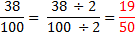Now we have a simplified fraction that represents 38%.

Example #2: 12%

Let's try another. 12% =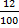Example #3: 12.5%

12.5% =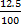=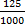We can get rid of the decimal by multiplying both the numerator and denominator by 10. Now we are ready to simplify the fraction. When faced with larger numbers, it is ok to use several steps when simplifying.=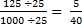This can be tougher if the percent is less than 1% or greater than 100%.

Example #4: 0.5%

0.5% =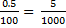=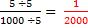Notice that in this example, we used the same idea for getting rid of the decimal. We just multiplied the top and bottom by ten to get 5 out of 1,000.

Example #5: 230%

230% ==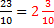When faced with a percent over 100%, you should either get a mixed number or improper fraction.We can use scale factors to help us convert from fractions to percents.

Example #6: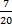We want to have a denominator of 100 so that we can easily write as a percent.
If we scale up the fraction by a factor of 5, we will have 20 x 5 =100 on the
denominator.= 35%

Example #7:When the denominator does not easily change to 100, you can convert to a decimal first. Then move the decimal over twice to the right to get the percent.= 0.375 =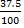= 37.5%

(In examples like this one, be sure not to reduce the fraction. You want the denominator to be 100 to convert to a percent.)

Example #8: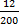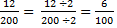= 6%

Example #9: 3When you have a mixed number, you can ignore the whole number until the end. We will focus on the four-fifths first. Then we will use the 3 to show that the percent will be over 300%. The whole number will go in the hundreds place in your percent.

3=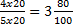= 380%

Notice that when the fraction is over 1, the percent is over 100%.

In conclusion, to convert percents, we need to still focus on the fact that percents are out of 100. Keeping this key idea in mind, we can convert to fractions by writing the fraction with a denominator of 100. Or if we start with the fraction, we can rewrite the fraction with a denominator of 100 or a decimal showing the hundredths place. Either way, the focus is still on percents being a part out of 100.

Math
Fractions
Decimals, Fractions and Percents Quiz
Math Vocabulary - Division and Fractions Quiz
Convert Decimals to Fractions Quiz

Multiply Fractions Quiz
Dividing Fractions Quiz
Factors